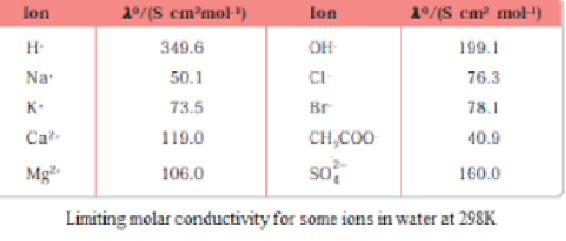# Kohlrausch law of independent migration of ions

According to Kohlrausch’s law, the equivalent conductivity of electrolyte on the infinite dilution is equal to total of conductance of both cations and anions.Equivalent limiting molar conductivity of the electrolyte refers to the algebraic sum of equivalent limiting molar conductivities of its constituent ions.Mathematically,Where,

λo+ = cations’ limiting equivalent conductivities

λo- = anions’ limiting equivalent conductivities

Uses of Kohlrausch’s law

• Degree of Dissociation’s calculation
• Molar Conductivity calculation at the infinite dilution in case of weak electrolytes
• For weak electrolyte, dissociation constant calculation
• Solubility calculation of Sparingly (infrequent, delicate, moderate) Soluble Salt

On the given concentration, solution’s molar conductivity is the conductance of solution’s volume which contains eletrolyte having one mole which is placed between the 2 electrodes having the cross-sections’ unit area and unit lenth distance. Solution’s molar conductivity rises up with decrement in concentration. This rise in molar conduct is due to the increase in total volumes which contains electrolyte having 1 mole.

Kohlrausch noticed definite regularities while doing the comparison of values of “limiting molar conductivities” of a few of the strong electrolytes. On the basis of his statement – Kohlrausch proposed that” “limiting molar conductivity of an electrolyte can be represented as the sum of the individual contributions of the anions and cations of the electrolyte”.

So, popularly this law is called the Kohlrausch law of independent migration of ions. For instance, limiting the molar conduction (Ëm°), sodium chloride’s Ëm° can be find out by having the appropriate knowledge of Sodium and chloride ions’ limiting the molar conductivities. A few of the significant Kohlrausch law’s applications of independent ion’s mifgration are:

1. Kohlrausch law helps in determining the limiting molar conductivities for any type of electrolyte ( weak and strong). Weak electrolytes consist of lower degree of dissociation and lower molar conductivities at high concentrations. As already seen in graph that for weak electrolyte, the line is not straight between c1/2 and molar conductivity; where c is concentration. Weak electrolyte’s molar conductivity increases rapidly at reduced concentrations. Thus, the limiting molar conductivity, Ëm° can’t be achieved by molar conducitvity’s extrapolation to ‘0’ cncentration. Therefore, usage of Kohlrausch law of independent migration of ions is there to determine limiting molar conductivity, Ëm°, considering weak electrolytes.
2. Also, Kohlrausch law help you to determine dissociation constant value from molar conductivity value and weak electrolyte having limiting molar conductivity at a known concentration.

α = ΛËm

Where, α = dissociation constant

Λ = molar conductivity

Ëm° = limiting molar conductivity